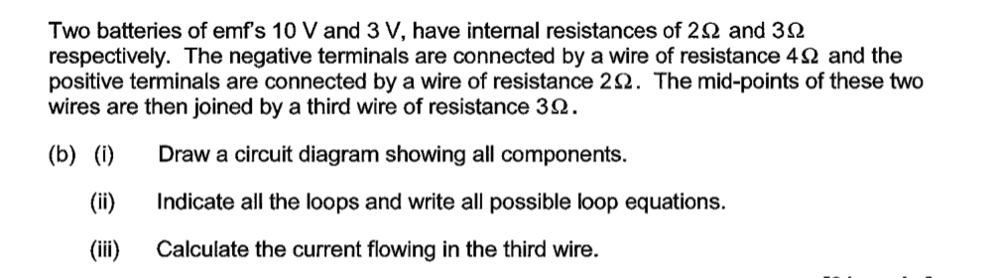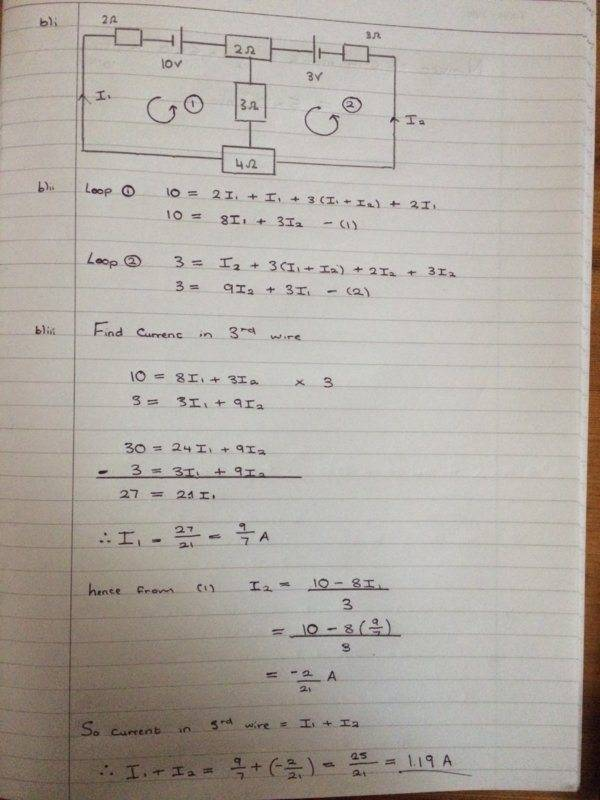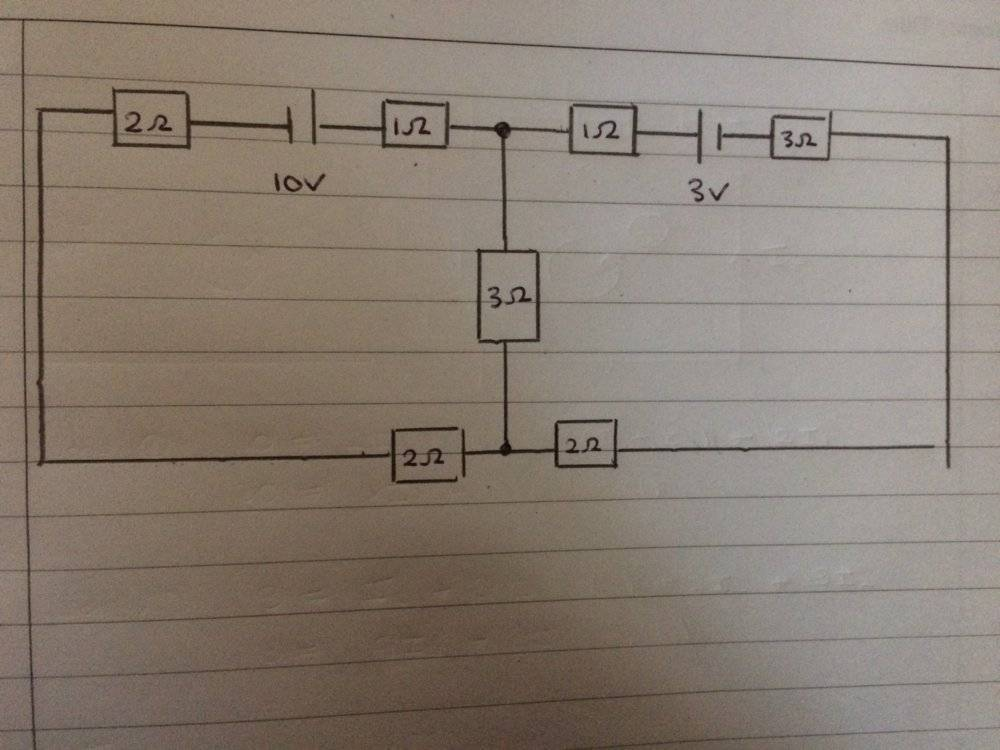# Using Kirchhoff's laws for multiple batteries and resistors

• Bolter

#### Bolter

Homework Statement
See full question below
Relevant Equations
Kirchhoff 1st and 2nd law
Hey everyone!

Had an attempt at this circuit question, but wanted to check if I got the set up right in order to find the current in the 3rd wire?Here is what I have doneThanks!

You have done well, showing the internal resistance in each battery as an explicit external resistor in series.

However, there is a problem with the depiction of the "mid-point" connections. The three ohm wire connects at the midpoints of the two ohm and the four ohm wires. It would be best to split the two ohm "resistor" at its midpoint and to do the same for the four ohm "resistor".

Because the drawing is misleading, the equations you constructed from it are not correct.

Last edited:
•Bolter
You have done well, showing the internal resistance in each battery as an explicit external resistor in series.

However, there is a problem with the depiction of the "mid-point" connections. The three ohm wire connects at the midpoints of the two ohm and the four ohm wires. It would be best to split the two ohm "resistor" at its midpoint and to do the same for the four ohm "resistor".

Because the drawing is misleading, the equations you constructed from it are not correct.

So would this be a much preferred circuit diagram then?I think my loop equations will be the same as before if I use Kirchhoff's law on left & right loop

•jbriggs444
•